# IP Protocol - Part 1: Binary & The Internet Protocol

To understand the Internet Protocol, we need to learn and understand Binary. It is very important to know and understand Binary because part of the IP protocol is also the "Subnetting" section which can only be explained and understood when an IP Address is converted to Binary!

This article deals with the analysis of IP addresses and covers the conversion of IP address to binary. We explain the conversion process with much detail using our well known diagrams. At the end of the article, readers will be able to understand and explain how IP address to binary conversion is performed and also calculate quickly the 32bit addressing scheme.

Those who are experienced in Binary can skim this section quickly, but do have a look through.

A lot of people are not aware that computers do not understand words, pictures and sounds, when we interact with them by playing a game, reading or drawing something on the screen. The truth is that all computers can understand is zeros (0) and ones (1) !

What we see on the screen is just an interpretation of what the computer understands, so the information displayed is useful and meaningful to us.

## Binary: Bits and Bytes

Everyone who uses the Internet would have, at one stage or another, come across the "Byte" or "Bit" term, usually when you're downloading, you get the speed indication in bytes or KBytes per second. We are going to see exactly what a Bit, Byte and KByte is, so you understand the terms.

To put it as simply as possible, a Bit is the smallest unit/value of Binary notation. The same way we say 1 cent is the smallest amount of money you can have , a Bit is the same thing but not in cents or dollars, but in Binary.

A Bit can have only one value, either a one (1) or a zero (0). So If I gave you a value of zero: 0, then you would say that is one Bit. If I gave you two of them: 00, you would say that's two Bits.

Now, if you had 8 zeros or ones together: 0110 1010 (I put a space in between to make it easier for the eyes) you would say that's 8 Bits or, one Byte ! Yes that is correct, 8 Bits are equal to one Byte.

The picture below gives you some examples: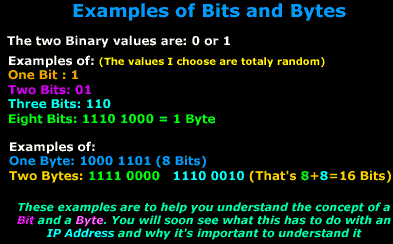It's like saying, if you have 100 cents, that is equal to one Dollar. In the same way, 8 Bits (doesn't matter if they are all 1s or 0s or a mixture of the two) would equal one Byte.

And to sum this all up, 1024 Bytes equal 1 KByte (Kilobyte). Why 1024 and not 1000 ? Well it's because of the way Binary works. If you did the maths, you would find the above correct.

## So what's Binary got to do with IP?

As explained during the introduction, computers display the zeros and ones in a way that makes the information useful to us. The Internet Protocol works a bit like this as well, where 98% of the time we see it in a decimal notation, but the computer understands it in binary. The picture below gives you an example of how a computer understands an IP Address: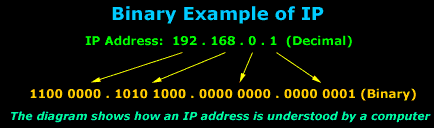The above example shows an IP address in decimal notation, which we understand more easily, this IP Address - 192.168.0.1 is then converted to Binary, which is what the computer understands and you can see how big the number gets ! It's easier for us to remember 4 different numbers than 32 zeros or ones !

Now, keeping in mind what we said earlier about Bits and Bytes, have you ever heard or read people saying that an IP Address is a 32 Bit address ? It is, and you can now see why: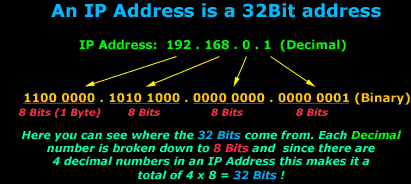So to sum up all the above, we now know what Binary notation is, what a Bit, Byte and KByte is and how Binary relates to an IP Address which is usally represented in its Decimal notation.

## Understanding the conversion between Decimal and Binary

Now we're going to look at how the conversion works between Decimal and Binary. This is an important step, because you'll probably find yourself in need of such a conversion when dealing with complex subnets.

The conversion is not that hard once you grasp the concept. The picture below shows an IP Address that we are going to convert to Binary. Keep in mind that the method I'm going to show you is the same for all conversions.

We are now going to convert the first octet in the IP Address 192.168.0.1 (Decimal) to Binary, in other words, we take the "192" and convert it to Binary and we are not going to have to do any difficult calculations, just simple additions:If you have read and understood the first section of this page, you should know that we need 8 bits to create one octet or, if you like, the 192 number. Each bit takes a certain value which never changes, this value is shown in purple, right above the bit, we then select the bits we need in such a way that the sum of all selected bits gives us the decimal number we need.

If you wanted to explain the conversion in mathematical terms, you would say that each bit is a power of 2 (2^), for example, bit 8 is actually '2^7' = 128 in decimal, bit 7 is '2^6 = 64 in decimal, bit 6 is '2^5' = 32 in decimal, bit 5 is '2^4' = 16 in decimal, bit 4 is '2^3' = 8 in decimal, bit 3 is '2^2' = 4 in decimal, bit 2 is '2^1' = 2 in decimal, bit 1 is '2^0' = 1 in decimal.

Note: When calculating the decimal value of an octet (192 in the example above), the Bit numbers do NOT represent the power of two value we must use in order to get the decimal value. This means that Bit 1 does NOT translate to 2^1=1 in decimal.

In our example, we used the 192. As you saw, we needed bits 8 and 7 and this gave us the Binary number of 11000000 which is 192 in Decimal. You must remember that the values of each bit never change! For example, bit 8 always has a decimal value of 128, whereas bit 1 always takes the value of 1. Using this method, you will find it easy to convert Decimal to Binary without the need for complex mathematical calculations.

So let's have a look at the next octet, which is the decimal number 168: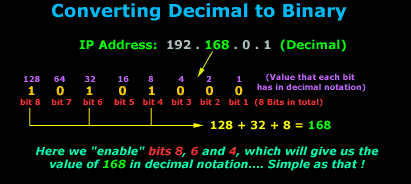Here again you can see that we needed to choose bits 8, 6 and 4 (in other words put a "1" in the bit's position) in order to get a decimal value of 168. So the Binary value of 10101000 is equal to the decimal value of 168.

Let's now look at all 4 octets of our IP Address, in Binary: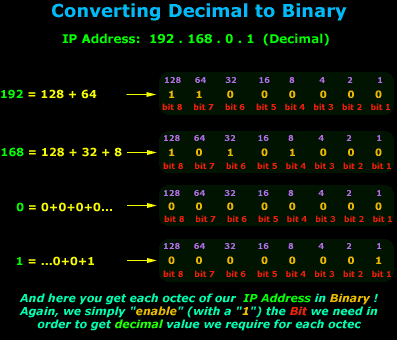No matter which way you convert, from Decimal to Binary or Binary to Decimal, the same method is used so you if you understood the above you should be able to convert either way any Binary or Decimal number.

That just about does it for this section, you're now ready for the next section that focuses on the Internet Protocol header.

44.200.101.84

### Wi-Fi Key Generator

Generate/Crack any
WEP, WPA, WPA2 Key!